Share

NCERT solutions for Class 12 Physics chapter 6 - Electromagnetic Induction

Physics Textbook for Class 12

NCERT Physics Class 12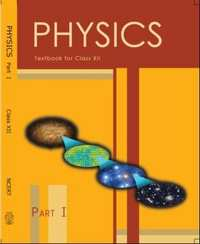Chapter 6: Electromagnetic Induction

Chapter 6: Electromagnetic Induction solutions [Pages 229 - 232]

Q 1 | Page 229

Predict the direction of induced current in the situations described by the following Figs. 6.18(a) to (f ).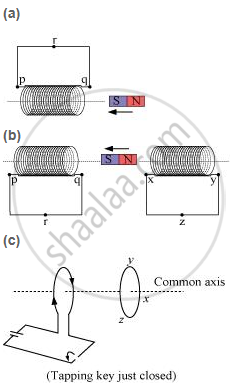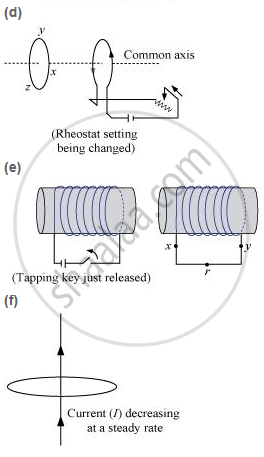Q 2 | Page 230

Use Lenz’s law to determine the direction of induced current in the situations described by Fig. 6.19:

(a) A wire of irregular shape turning into a circular shape;

(b) A circular loop being deformed into a narrow straight wire.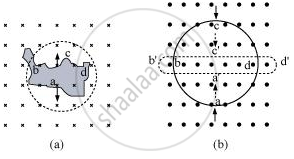Q 3 | Page 230

A long solenoid with 15 turns per cm has a small loop of area 2.0 cmplaced inside the solenoid normal to its axis. If the current carried by the solenoid changes steadily from 2.0 A to 4.0 A in 0.1 s, what is the induced emf in the loop while the current is changing?

Q 4 | Page 230

A rectangular wire loop of sides 8 cm and 2 cm with a small cut is moving out of a region of uniform magnetic field of magnitude 0.3 T directed normal to the loop. What is the emf developed across the cut if the velocity of the loop is 1 cm s−1 in a direction normal to the (a) longer side, (b) shorter side of the loop? For how long does the induced voltage last in each case?

Q 5 | Page 230

A 1.0 m long metallic rod is rotated with an angular frequency of 400 rad s−1 about an axis normal to the rod passing through its one end. The other end of the rod is in contact with a circular metallic ring. A constant and uniform magnetic field of 0.5 T parallel to the axis exists everywhere. Calculate the emf developed between the centre and the ring.

Q 6 | Page 230

A circular coil of radius 8.0 cm and 20 turns is rotated about its vertical diameter with an angular speed of 50 rad s−1 in a uniform horizontal magnetic field of magnitude 3.0×10−2 T. Obtain the maximum and average emf induced in the coil. If the coil forms a closed loop of resistance 10Ω, calculate the maximum value of current in the coil. Calculate the average power loss due to Joule heating. Where does this power come from?

Q 7 | Page 230

A horizontal straight wire 10 m long extending from east to west is falling with a speed of 5.0 m s−1, at right angles to the horizontal component of the earth’s magnetic field, 0.30 × 10−4 Wb m−2.

(a) What is the instantaneous value of the emf induced in the wire?

(b) What is the direction of the emf?

(c) Which end of the wire is at the higher electrical potential?

Q 8 | Page 230

Current in a circuit falls from 5.0 A to 0.0 A in 0.1 s. If an average emf of 200 V induced, give an estimate of the self-inductance of the circuit.

Q 9 | Page 230

A pair of adjacent coils has a mutual inductance of 1.5 H. If the current in one coil changes from 0 to 20 A in 0.5 s, what is the change of flux linkage with the other coil?

Q 10 | Page 230

A jet plane is travelling towards west at a speed of 1800 km/h. What is the voltage difference developed between the ends of the wing having a span of 25 m, if the Earth’s magnetic field at the location has a magnitude of 5 × 10−4 T and the dip angle is 30°.

Q 11 | Page 231

Suppose the loop in Exercise 6.4 is stationary but the current feeding the electromagnet that produces the magnetic field is gradually reduced so that the field decreases from its initial value of 0.3 T at the rate of 0.02 T s−1. If the cut is joined and the loop has a resistance of 1.6 Ω how much power is dissipated by the loop as heat? What is the source of this power?

Q 12 | Page 231

A square loop of side 12 cm with its sides parallel to X and Y axes is moved with a velocity of 8 cm s−1in the positive x-direction in an environment containing a magnetic field in the positive z-direction. The field is neither uniform in space nor constant in time. It has a gradient of 10−3 T cm−1 along the negative x-direction (that is it increases by 10− 3 T cm−1 as one moves in the negative x-direction), and it is decreasing in time at the rate of 10−3 T s−1. Determine the direction and magnitude of the induced current in the loop if its resistance is 4.50 mΩ.

Q 13 | Page 231

It is desired to measure the magnitude of field between the poles of a powerful loud speaker magnet. A small flat search coil of area 2 cm2 with 25 closely wound turns, is positioned normal to the field direction, and then quickly snatched out of the field region. Equivalently, one can give it a quick 90° turn to bring its plane parallel to the field direction). The total charge flown in the coil (measured by a ballistic galvanometer connected to coil) is 7.5 mC. The combined resistance of the coil and the galvanometer is 0.50 Ω. Estimate the field strength of magnet.

Q 14 | Page 231

Figure 6.20 shows a metal rod PQ resting on the smooth rails AB and positioned between the poles of a permanent magnet. The rails, the rod, and the magnetic field are in three mutual perpendicular directions. A galvanometer G connects the rails through a switch K. Length of the rod = 15 cm, = 0.50 T, resistance of the closed loop containing the rod = 9.0 mΩ. Assume the field to be uniform.

(a) Suppose K is open and the rod is moved with a speed of 12 cm s−1 in the direction shown. Give the polarity and magnitude of the induced emf.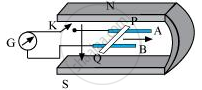(b) Is there an excess charge built up at the ends of the rods when

K is open? What if K is closed?

(c) With K open and the rod moving uniformly, there is no net force on the electrons in the rod PQ even though they do experience magnetic force due to the motion of the rod. Explain.

(d) What is the retarding force on the rod when K is closed?

(e) How much power is required (by an external agent) to keep the rod moving at the same speed (=12 cm s−1) when K is closed? How much power is required when K is open?

(f) How much power is dissipated as heat in the closed circuit?

What is the source of this power?

(g) What is the induced emf in the moving rod if the magnetic field is parallel to the rails instead of being perpendicular?

Q 15 | Page 232

An air-cored solenoid with length 30 cm, area of cross-section 25 cm2 and number of turns 500, carries a current of 2.5 A. The current is suddenly switched off in a brief time of 10−3 s. How much is the average back emf induced across the ends of the open switch in the circuit? Ignore the variation in magnetic field near the ends of the solenoid.

Q 16 | Page 232

(a) Obtain an expression for the mutual inductance between a long straight wire and a square loop of side as shown in Fig. 6.21.

(b) Now assume that the straight wire carries a current of 50 A and the loop is moved to the right with a constant velocity, = 10 m/s.

Calculate the induced emf in the loop at the instant when = 0.2 m.

Take = 0.1 m and assume that the loop has a large resistance.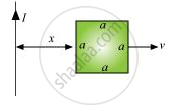Q 17 | Page 232

A line charge λ per unit length is lodged uniformly onto the rim of a wheel of mass and radius R. The wheel has light non-conducting spokes and is free to rotate without friction about its axis (Fig. 6.22). A uniform magnetic field extends over a circular region within the rim. It is given by,

= − B0 (≤ aR)

= 0 (otherwise)

What is the angular velocity of the wheel after the field is suddenly switched off?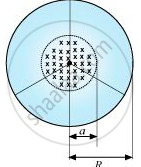Chapter 6: Electromagnetic Induction

NCERT Physics Class 12NCERT solutions for Class 12 Physics chapter 6 - Electromagnetic Induction

NCERT solutions for Class 12 Physics chapter 6 (Electromagnetic Induction) include all questions with solution and detail explanation. This will clear students doubts about any question and improve application skills while preparing for board exams. The detailed, step-by-step solutions will help you understand the concepts better and clear your confusions, if any. Shaalaa.com has the CBSE Physics Textbook for Class 12 solutions in a manner that help students grasp basic concepts better and faster.

Further, we at Shaalaa.com are providing such solutions so that students can prepare for written exams. NCERT textbook solutions can be a core help for self-study and acts as a perfect self-help guidance for students.

Concepts covered in Class 12 Physics chapter 6 Electromagnetic Induction are Electromagnetic Induction Questions, Ac Generator, Self-Inductance, Energy Consideration: a Quantitative Study, Motional Electromotive Force, The Experiments of Faraday and Henry, Magnetic Flux, Faraday’s Law of Induction, Mutual Inductance, Eddy Currents, Lenz’S Law and Conservation of Energy, Induced Emf and Current, Electromagnetic Induction.

Using NCERT Class 12 solutions Electromagnetic Induction exercise by students are an easy way to prepare for the exams, as they involve solutions arranged chapter-wise also page wise. The questions involved in NCERT Solutions are important questions that can be asked in the final exam. Maximum students of CBSE Class 12 prefer NCERT Textbook Solutions to score more in exam.

Get the free view of chapter 6 Electromagnetic Induction Class 12 extra questions for Physics and can use Shaalaa.com to keep it handy for your exam preparation

S